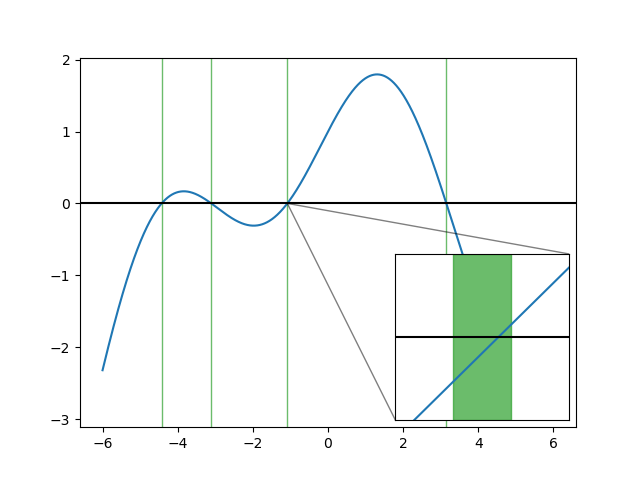89

53

24

15

# IntervalRootFinding.jlThis package provides guaranteed methods for finding roots of functions, i.e. solutions to the equation `f(x) == 0` for a function `f`. To do so, it uses methods from interval analysis, using interval arithmetic from the `IntervalArithmetic.jl` package by the same authors.

## Basic usage examples

The basic function is `roots`. A standard Julia function and an interval is provided and the `roots` function return a list of intervals containing all roots of the function located in the starting interval.

``````julia> using IntervalArithmetic, IntervalRootFinding

julia> f(x) = sin(x) - 0.1*x^2 + 1
f (generic function with 1 method)

julia> roots(f, -10..10)
4-element Array{Root{Interval{Float64}},1}:
Root([3.14959, 3.1496], :unique)
Root([-4.42654, -4.42653], :unique)
Root([-1.08205, -1.08204], :unique)
Root([-3.10682, -3.10681], :unique)
``````

The `:unique` status tell us, in addition, that each listed region contains exactly one root. The other possible status is `:unknown`, which corresponds to intervals that may contain zero, one, or more roots - no guarantee is provided for these intervals.

These results are represented in the following plot, the region containing roots being in green. The inset show a close-up of one of the roots:The full documentation is available here.

## Authors

• Luis Benet, Instituto de Ciencias Físicas, Universidad Nacional Autónoma de México (UNAM)
• David P. Sanders, Departamento de Física, Facultad de Ciencias, Universidad Nacional Autónoma de México (UNAM)

## Acknowledgements

Financial support is acknowledged from DGAPA-UNAM PAPIME grants PE-105911 and PE-107114, and DGAPA-UNAM PAPIIT grants IN-117214 and IN-117117. LB acknowledges support through a Cátedra Moshinsky (2013).

04/02/2017

7 days ago

186 commits